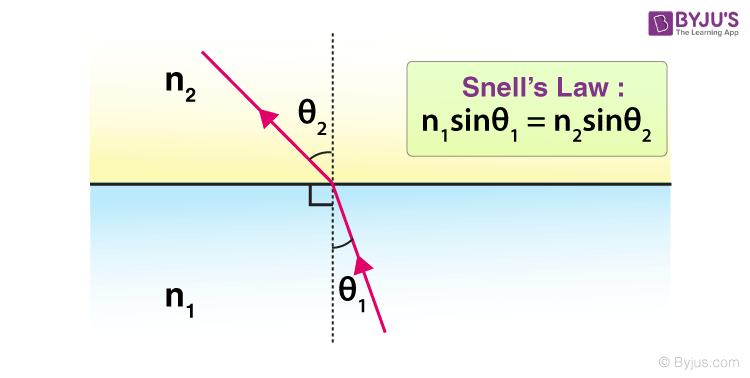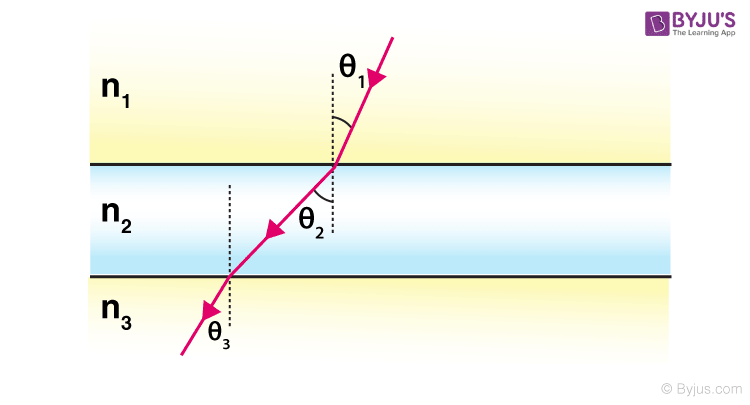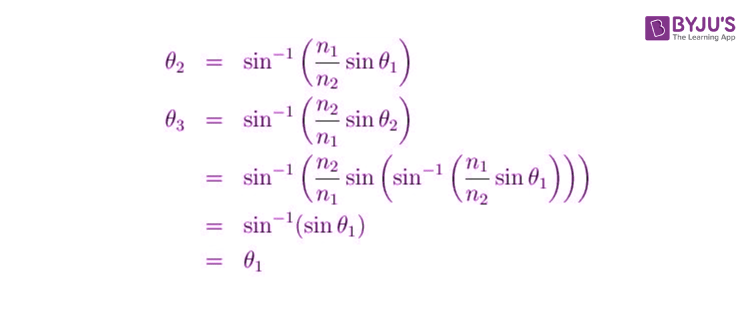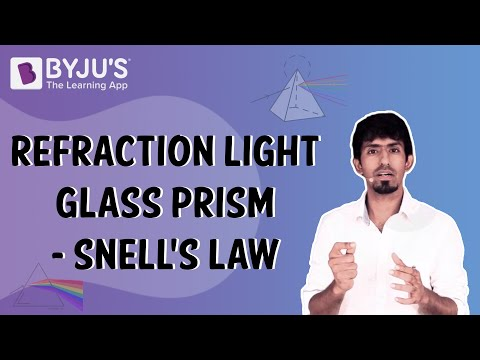Checkout JEE MAINS 2022 Question Paper Analysis : Checkout JEE MAINS 2022 Question Paper Analysis :

# The Law of Refraction - Snell's Law

Discovered by Willebrord Snell in 1621 the laws of refraction are also termed as Snell’s law. As we know the refraction or bending of light takes place when it travels from medium to medium. The degree of bend is predicted by the help of this law. To comprehensively understand a discussion on lenses and their application one must be familiar with the concepts of refraction.

## Snell’s Law – The Laws of Refraction

The point of refraction is created where the incident rays lands and the angle that it makes with the refracted ray not forgetting the normal line that is dropped on the plane perpendicularly. The medium through which the rays of light are passing creates a considerable difference in refraction unlike in reflection of light. The refractive indices make the dependency on the medium apparent in Snell’s Law.

The following is a diagrammatic representation:The normal on the surface is used to gauge the angles that the refracted ray creates at the contact point. n1 and n2 are the two different mediums that will impact the refraction.

The refractive index of water is 1.33 whereas the refractive index of air is 1.00029. Thus, to understand the concept of Snell’s Law let’s consider the light of wavelength 600 nm that goes from water into the air. To calculate the angle made by the outgoing ray we apply the figures in the formula mentioned above.

1.33 sin 30o = 1.00029 sin x

x = 41o

When one fishes with a spear it is not as difficult as fishing with a rod as the fisherman has to encounter refraction in the latter case.

### Complex Snell’s Law Diagram

A complex diagram of Snell’s Law displays something that is not directly obvious. A ray of light passes through the glass and standing behind it the viewer experiences refraction through three media. The situation is represented in the following diagram.What we see in this is that the light ray incoming is parallel to the outgoing light ray.

As the angle of incidence (i) and angle of refraction (r) thus rearranging Snell’s Law:

• n1sin(i) = n2sin(r)
• (n1/n2)sin(i) = sin(r)

This gives us a qualitative representation of refraction. Thus, we’ll use this equation to understand the concept of multiple refractions. When moving from a section of the high index to a region of a low index, the ray will refract away from the normal. When the ray moves from a section of the lower index to the higher index the ray of incidence will bend towards the normal.

## Watch the video to visualise the refraction of light through a glass prism.Test your knowledge on Law of refraction